# Interpreting Predictive Models Using Partial Dependence Plots

#### 2021-05-28

Despite their historical and conceptual importance, linear regression models often perform poorly relative to newer predictive modeling approaches from the machine learning literature like support vector machines, gradient boosting machines, or random forests. An objection frequently leveled at these newer model types is difficulty of interpretation relative to linear regression models, but partial dependence plots may be viewed as a graphical representation of linear regression model coefficients that extends to arbitrary model types, addressing a significant component of this objection. The DataRobot modeling engine is a massively parallel architecture for simultaneously fitting many models to a single dataset, providing a basis for comparing these models and selecting the most appropriate one for use, by any one of several different criteria. These models cover a wide range of types, including constrained and unconstrained linear regression models, machine learning models like the ones listed above, and ensemble models that combine the predictions of the most successful of these individual models in several different ways. This vignette illustrates the use of partial dependence plots to characterize the behavior of four very different models, all developed to predict the compressive strength of concrete from the measured properties of laboratory samples. These plots are based on predictions generated by the R package datarobot, which allows interactive R users to start new DataRobot modeling projects, collect the results, and generate predictions for any of these models from any dataset that is compatible with the original modeling dataset. A more detailed introduction to this R package is given in the companion vignette, “Introduction to the DataRobot R Package.”

## 1. Introduction

The DataRobot modeling engine is a commercial product that supports the rapid development and evaluation of a large number of different predictive models from a single data source. The open-source R package datarobot allows users of the DataRobot modeling engine to interact with it from R, creating new modeling projects, examining model characteristics, and generating predictions from any of these models for a specified dataset. This vignette describes an application of the datarobot package, demonstrating how R can be used to apply novel preprocessing of the DataRobot modeling data to obtain useful new insights. More specifically, Section 2 describes the creation of a DataRobot modeling project to predict concrete compressive strength from the characteristics of laboratory material samples. Modified modeling datasets are then used with the datarobot prediction functions to generate partial dependence plots, described in Section 3. These plots, proposed by Friedman (2001) as a useful adjunct to gradient boosting machines, can be constructed for any predictive model and they provide useful insight into the way model predictions depend on covariates, as shown in Section 4. Conceptually, these plots may be viewed as graphical extensions of simple linear regression model parameters to arbitrarily complex models without useful parametric descriptions.

## 2. Example: compressive strength of concrete

As noted by Boukhatem et al. (2012), concrete is a composite material widely used in the construction industry, typically consisting of cement, water, coarse aggregates, fine aggregates, and possibly other components like blast furnace slag, fly ash, silica fume, superplaticizers and air entraining agents. In fact, Chandwani, Agrawal, and Nagar (2014) characterize concrete as “one of the most widely used construction materials in the world today,” further noting that:

The material modeling of concrete is a difficult task owing to its composite nature. Although various empirical relationships in the form of regression equations have been derived from experimental results and are widely in use, these do not provide accuracy and predictability wherein the interactions among the number of variables are either unknown, complex, or nonlinear in nature.’’

To address these difficulties, Chandwani, Agrawal and Nagar explore the use of artificial neural networks as a more effective alternative to linear regression models. The following example considers a wider range of predictive model structures that includes a linear regression model and several machine learning models, both to demonstrate how much better some of these other models can perform, and to illustrate the use of the partial dependence plots advocated by Friedman (2001) in understanding the behavior of relatively complex models.

The results presented here are based on a concrete physical properties dataset provided by Yeh (1998) and available from a number of different sources. The version used here was constructed from the dataframe ConcreteCompressiveStrength, included in the R package randomUniformForest (Ciss 2015). This dataframe characterizes 1030 laboratory concrete samples, giving the compressive strength and age of each sample, along with seven compositional variables. Several of the variable names in this dataframe include embedded spaces, a potential source of subtle errors in some data manipulations, motivating the slightly modified dataframe considered here. As a specific example, the StartProject and UploadPredictionDataset functions in the datarobot package can both accept either dataframes or CSV files, but dataframes are first saved by these functions as CSV files, which are then uploaded to the modeling engine. If we write the dataframe ConcreteCompressiveStrength to a CSV file using R’s write.csv function, the variable names are changed, with periods replacing the spaces. This mismatch in variable names between the dataframe and the CSV file can cause difficulties if we attempt to compare predictions from the DataRobot models with observed responses from the original dataframe. To avoid these problems, the analysis presented here is based on the dataframe concreteFrame, identical to ConcreteCompressiveStrength except with the following, somewhat simplified variable names:

str(concreteFrame)
## 'data.frame':    1030 obs. of  9 variables:
##  $cement : num 540 540 332 332 199 ... ##$ blastFurnaceSlag: num  0 0 142 142 132 ...
##  $flyAsh : num 0 0 0 0 0 0 0 0 0 0 ... ##$ water           : num  162 162 228 228 192 228 228 228 228 228 ...
##  $superplasticizer: num 2.5 2.5 0 0 0 0 0 0 0 0 ... ##$ coarseAggregate : num  1040 1055 932 932 978 ...
##  $fineAggregate : num 676 676 594 594 826 ... ##$ age             : int  28 28 270 365 360 90 365 28 28 28 ...
##  $strength : num 80 61.9 40.3 41 44.3 ... This dataframe was used to create a DataRobot project as described in the vignette, “Introduction to the DataRobot R Package.” The specific sequence used here was: myDRProject <- StartProject(concreteFrame, "ConcreteProject", target = "strength", wait = TRUE) Once the modeling process has completed, the ListModels function returns an S3 object of class “listOfModels” that characterizes all of the models in a specified DataRobot project. concreteModels <- ListModels(myDRProject) A summary method has been included in the datarobot package for “listOfModels” objects that gives a useful preview of the models included in a DataRobot modeling project: summary(concreteModels) ##$generalSummary
##  "First 6 of 30 models from: concreteModels (S3 object of class listOfModels)"
##
## $detailedSummary ## modelType ## 1 ENET Blender ## 2 Advanced AVG Blender ## 3 AVG Blender ## 4 ENET Blender ## 5 Gradient Boosted Greedy Trees Regressor (Least-Squares Loss) ## 6 Gradient Boosted Trees Regressor (Least-Squares Loss) ## expandedModel ## 1 ENET Blender ## 2 Advanced AVG Blender ## 3 AVG Blender ## 4 ENET Blender ## 5 Gradient Boosted Greedy Trees Regressor (Least-Squares Loss)::Tree-based Algorithm Preprocessing v15 ## 6 Gradient Boosted Trees Regressor (Least-Squares Loss)::Tree-based Algorithm Preprocessing v2 ## modelId blueprintId ## 1 56efefd61f0d8d324c6f5b6b be994f177e7682acdb2d27990ddb235e ## 2 56efefd61f0d8d324c6f5b67 3a1d4bf173ea4836b12d7210017a1e54 ## 3 56efefd61f0d8d324c6f5b63 1558d9bf22204fc7fe3d4e8d92084f70 ## 4 56efefd61f0d8d324c6f5b6f e3e3b7f9201353e3d2c4dff90ecd2a99 ## 5 56efeecf905090323586004a 45e6a473a324351a7867af171fd22983 ## 6 56efeecf9050903235860049 ecdaf36f60a0c2487f306e24fc48a4f1 ## featurelistName featurelistId samplePct validationMetric ## 1 Informative Features 56efeec51f0d8d32236f5b68 63.9806 4.06815 ## 2 Informative Features 56efeec51f0d8d32236f5b68 63.9806 4.10597 ## 3 Informative Features 56efeec51f0d8d32236f5b68 63.9806 4.13710 ## 4 Informative Features 56efeec51f0d8d32236f5b68 63.9806 4.13884 ## 5 Informative Features 56efeec51f0d8d32236f5b68 63.9806 4.17213 ## 6 Informative Features 56efeec51f0d8d32236f5b68 63.9806 4.28405 The first element of this summary list, named generalSummary, tells us how many models are included in the concreteModels list, and the second element, named detailedSummary, contains the first 6 rows of the dataframe obtained from the as.data.frame method with its default parameters. For “listOfModels” objects, this method converts concreteModels into a dataframe with one row for each project model and the following columns: • modelType = a (relatively) short character string describing the model structure; • expandedModel = the modelType character string, prepended with character strings describing the preprocessing steps applied, if any; • modelId = unique alphanumeric model identifier; • blueprintId = unique alphanumeric identifier for the DataRobot blueprint used in fitting the model: this identifier defines the structure of the model and its preprocessing; • featurelistName = character string giving the name of the featurelist containing the features (e.g., columns of the modeling dataset) used in fitting all project models; • featurelistId = unique alphanumeric identifier for the featurelist; • samplePct = percentage of the training dataset used to fit the model; • validationMetric = value of the metric used in fitting all project models, evaluated for the validation dataset. Each element of the original S3 “listOfModels” object is itself an S3 object, of class “dataRobotModel,” with its own summary method; these objects contain more model information than is included in the dataframe constructed above. Complete results can be obtained by calling the function as.data.frame with simple = FALSE. For the concrete modeling project considered here, the resulting dataframe has 48 columns, versus the 8 described above.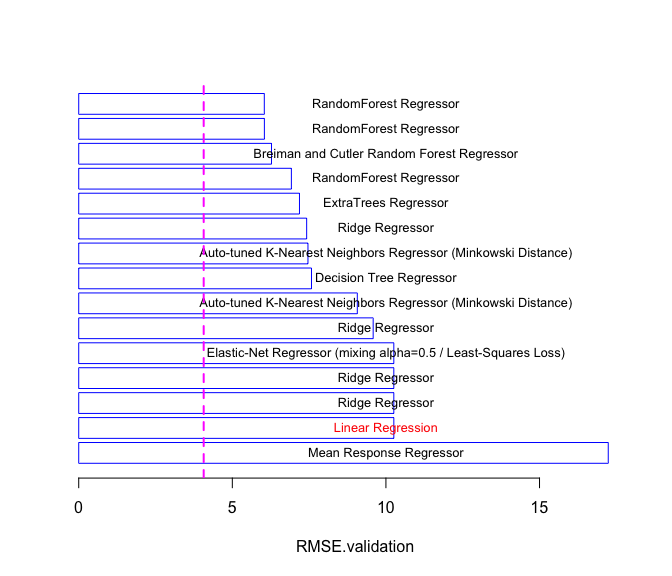Figure 1: Validation set performance for the 15 poorer-performing predictive models. Figures 1 and 2 are barplots summarizing the performance of the concrete predictive models, based on the RMSE values from concreteModels. This project includes 30 models and, to make the details easier to see, each figure summarizes half of these models, with Figure 1 summarizing the 15 poorer-performing models and Figure 2 summarizing the 15 better-performing models. These plots were generated using the plot method included in the datarobot package for S3 objects of class “listOfModels.” This method generates a horizontal barplot where the length of each bar is determined by the optional parameter metric and the text displayed on each bar in the plot is determined by the modelType value for each model. The default value for metric is NULL, which specifies that the validationMetric value from the summary dataframe is used, as in this example. The only required parameter is the S3 object; the four optional parameters used in this example have the following effects: • orderDecreasing, when TRUE, sorts the models in decreasing order of metric. Here, since metric has the default value NULL, models are sorted by decreasing validationMetric value, which is RMSE.validation for this example; • selectRecords is a numeric vector specifying a subset of models to be included in the plot. In Figure 1, the poorer half of the models (ranked 16 through 30) are selected; • textColor is a character vector of color names for the text labels in each barplot; • xpos is a numerical value defining the horizontal center position of the text labels; • xlim is passed to R’s barplot function to specify the horizontal extent of the plot. For a more detailed discussion of this plot method and its options, refer to the help file. In both Figures 1 and 2, the vertical dashed line indicates the performance achieved by the ENET Blender discussed below, which exhibits the best overall performance. The poorest model shown in Figure 1 is the Mean Response Regressor, a trivial, intercept-only model included as a performance reference for all of the other models in the project. The project includes one unconstrained linear regression model (Linear Regression, indicated in red), and its performance is part of a four-way tie for second-worst place, together with three constrained linear regression models: two ridge regression models and one elastic net model. The difference between the two ridge regression models - and between these models and the other two, better-performing ridge regression models in the project - lies in their preprocessing. While this information is not shown in Figure 1, it is available from the expandedModel field of the project model list described above. For the four ridge regression models, we have the following results: ridgeRows <- grep("Ridge", modelsFrame$modelType)
modelsFrame[ridgeRows, c("expandedModel", "validationMetric")]
##                                                  expandedModel validationMetric
## 21                           Ridge Regressor::Constant Splines          7.41968
## 25 Ridge Regressor::Regularized Linear Model Preprocessing v14          9.58482
## 26  Ridge Regressor::Regularized Linear Model Preprocessing v1         10.25682
## 27        Ridge Regressor::Missing Values Imputed::Standardize         10.25682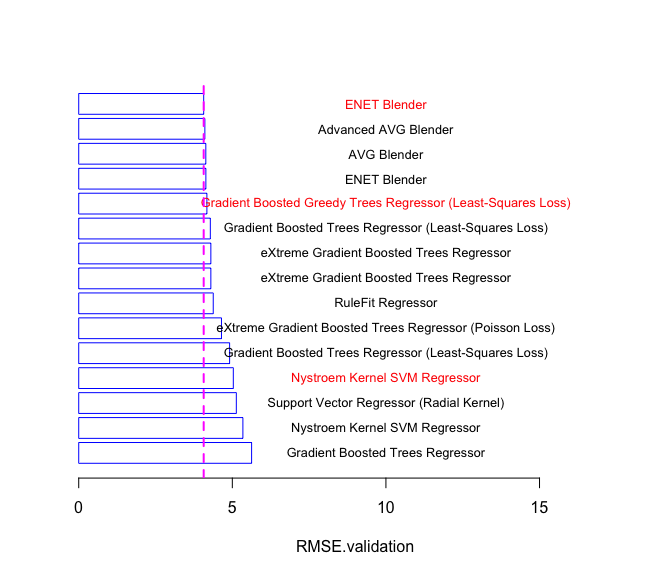Figure 2: Validation set performance for the 15 better-performing predictive models.

Figure 2 summarizes the performance of the other 15 models from the concrete compressive strength prediction project, in the same format as Figure 1. The four best models in the project appear at the top of this plot and are blenders, composite models that aggregate two or more of the best individual models from the project, differing in which of these models are included and how they are combined. It is clear from Figure 2 that these blenders exhibit essentially identical performance. The best performance is achieved by the ENET Blender, shown in red in Figure 2; two other models are also shown in red: Gradient Boosted Greedy Trees Regressor, the best non-blender model, and the better-performing of two Nystroem Kernel SVM Regressor models. As with the ridge regression models discussed above, the difference between these SVM models lies in the preprocessing applied in fitting each one. The partial dependence plots described in Section 3 are used in Section 4 to obtain insights into the performance differences between the four models highlighted in red in Figures 1 and 2.

## 3. Partial dependence plots

In the case of linear regression, we can gain considerable insight into the structure and interpretation of the model by examining its coefficients. For more complex models like support vector machines, random forests, or the blenders considered here, no comparably simple parametric description is available, making the interpretation of these models more difficult. To address this difficulty for his gradient boosting machine, Friedman (2001) proposed the use of partial dependence plots. The rest of this section briefly describes these plots, and the following section demonstrates their use in interpreting the four models highlighted in red in Figures 1 and 2.

Friedman’s partial dependence plots are based on the following idea: consider an arbitrary model obtained by fitting a particular structure (e.g., random forest, support vector machine, or linear regression model) to a given dataset $$\cal D$$. This dataset includes $$N$$ observations $$y_k$$ of a response variable $$y$$, for $$k = 1,2,\dots,N$$, along with $$p$$ covariates denoted $$x_{i,k}$$ for $$i = 1,2,\dots,p$$ and $$k = 1,2,\dots,N$$. The model generates predictions of the form:

$\hat{y}_k = F(x_{1,k}, x_{2,k}, \dots, x_{p,k}),$

for some mathematical function $$F(\cdots)$$. In the case of a single covariate $$x_j$$, Friedman’s partial dependence plots are obtained by computing the following average and plotting it over a useful range of $$x$$ values:

$\phi_j(x) = \frac{1}{N} \; \sum_{k=1}^{N} \; F(x_{1,k},\dots, x_{j-1,k},x,x_{j+1,k}, \dots, x_{p,k}).$

The idea is that the function $$\phi_j(x)$$ tells us how the value of the variable $$x_j$$ influences the model predictions $$\{ \hat{y}_k \}$$ after we have “averaged out” the influence of all other variables. For linear regression models, the resulting plots are simply straight lines whose slopes are equal to the model parameters. Specifically, for a linear model, the prediction defined above has the form:

$\hat{y}_k = \sum_{i=1}^{p} \; a_i x_{i,k},$

from which it follows that the partial dependence function is:

$\phi_j(x) = a_j x + \frac{1}{N} \; \sum_{k=1}^{N} \sum_{i \neq j} \; a_i x_{i,k} = a_j x + \sum_{i \neq j} a_i \bar{x_i},$

where $$\bar{x_i}$$ is the average value of the $$i^{th}$$ covariate. The main advantage of these plots is that they can be constructed for any predictive model, regardless of its form or complexity.

The multivariate extension of the partial dependence plots just described is straightforward in principle, but several practical issues arise. First and most obviously, these plots are harder to interpret: the bivariate partial dependence function $$\phi_{i,j}(x,y)$$ for two covariates $$x_i$$ and $$x_j$$ is defined analogously to $$\phi(x)$$ by averaging over all other covariates, and this function is still relatively easy to plot and visualize, but higher-dimensional extensions are problematic. Also, these multivariate partial dependence plots have been criticized as being inadequate in the face of certain strong interactions; an alternative approach that attempts to address these limitations has been incorporated into the R package ICEbox described by Goldstein et al. (2015). It may be useful to extend the approach described here along the lines advocated by these authors, but this idea has not yet been explored.

## 4. Results for the concrete models

To gain an understanding of the differences between some of the models characterized in Figures 1 and 2, the following paragraphs construct the univariate partial dependence plots described in Section 3 for selected variables and the four models highlighted in Section 2:

1. ENET Blender, the model exhibiting the best performance seen for this example;
2. GBGT: Gradient Boosted Greedy Trees Regressor, the best non-blender model;
3. Nystroem Kernel SVM: the best of the support vector machine (SVM) models;
4. Linear: the ordinary least squares linear regression model.

To compute $$\phi_j(x)$$, we first select a set of $$G$$ grid points uniformly spaced over the range of $$x_j$$ values. Next, we construct an augmented dataset by concatenating $$G$$ copies of the original, each constructed by replacing all $$x_j$$ observations with one of the $$G$$ grid points. A simple R function to generate this augmented dataset is FullAverageDataset:

FullAverageDataset <- function(covarFrame, refCovar, numGrid, plotRange = NULL) {
covars <- colnames(covarFrame)
refIndex <- which(covars == refCovar)
refVar <- covarFrame[, refIndex]
if (is.null(plotRange)) {
start <- min(refVar)
end <- max(refVar)
} else {
start <- plotRange
end <- plotRange
}
grid <- seq(start, end, length = numGrid)
outFrame <- covarFrame
outFrame[, refIndex] <- grid
for (i in 2:numGrid) {
upFrame <- covarFrame
upFrame[, refIndex] <- grid[i]
outFrame <- rbind.data.frame(outFrame, upFrame)
}
outFrame
}

This function is called with a dataframe covarFrame containing covariates only, a character string refCovar giving the name of the reference covariate $$x_j$$ for which the partial dependence results are desired, and two optional parameters. The optional parameter numGrid specifies the number of grid points for which $$\phi_j(x)$$ is computed, and plotRange is an optional two-element vector that specifies the minimum and maximum $$x$$ values to be used; the default option, plotRange = NULL, uses the minimum and maximum $$x_j$$ values in the dataset.

In what follows, this function is used to create the modified dataset required to compute $$\phi_j(x)$$ for each covariate of interest, for the four models highlighted in red in Figures 1 and 2. This is accomplished via the function PDPbuilder shown here, which calls the function FullAverageDataset just described:

PDPbuilder <- function(covarFrame, refCovar, listOfModels,
numGrid = 100, plotRange = NULL) {
augmentedFrame <- FullAverageDataset(covarFrame, refCovar,
numGrid, plotRange)
nModels <- length(listOfModels)
library(doBy)
model <- listOfModels[]
yHat <- Predict(model, augmentedFrame)
hatFrame <- augmentedFrame
hatFrame$prediction <- yHat hatSum <- summaryBy(list(c("prediction"), c(refCovar)), data = hatFrame, FUN = mean) colnames(hatSum) <- model$modelType

for (i in 2:nModels) {
model <- listOfModels[[i]]
yHat <- Predict(model, augmentedFrame)
hatFrame <- augmentedFrame
hatFrame$prediction <- yHat upSum <- summaryBy(list(c("prediction"), c(refCovar)), data = hatFrame, FUN = mean) colnames(upSum) <- model$modelType
hatSum <- merge(hatSum, upSum)
}
hatSum
}

The first two required paramters and the two optional parameters for this function are the same as those for the FullAverageDataset function, and the only other parameter in the PDPbuilder call is listOfModels, which specifies the DataRobot models for which partial dependence results are to be computed. This function returns a dataframe whose first column contains the $$x_j$$ values of the grid points for which the partial dependence is evaluated, and subsequent columns contain the $$\phi_j(x)$$ values for each model in listOfModels at each of these grid points.

In the examples considered here, these models are the four appearing in red in Figures 1 and 2, corresponding to the models ranked 1 (ENET Blender), 5 (Gradient Boosted Greedy Trees Regressor (Least-Squares Loss)), 12 (Nystroem Kernel SVM Regressor), and 29 (Linear Regression). The following code generates this list of models and the associated partial dependence results for the age variable from the concrete modeling dataframe:

modelList <- list(concreteModels[], concreteModels[],
concreteModels[], concreteModels[])
agePDPframe <- PDPbuilder(concreteFrame[, 1:8], "age", modelList)

Given this dataframe, partial dependence plots like that shown in Figure 3 are generated by calling the plot function listed below:

PDPlot <- function(PDframe, Response, ltypes,
lColors, ...) {
Rng <- range(Response)
nModels <- ncol(PDframe) - 1
modelNames <- colnames(PDframe)[2: (nModels + 1)]
plot(PDframe[, 1], PDframe[, 2], ylim=Rng, type = "l",
lty = ltypes, lwd = 2, col = lColors,
xlab = colnames(PDframe),
ylab = "Partial Dependence", ...)
abline(h = Rng, lty=3, lwd=2, col="black")
for (i in 2:nModels) {
lines(PDframe[, 1], PDframe[, i + 1], lwd = 2,
lty = ltypes[i], col = lColors[i])
}
legend("topleft", lty = ltypes, text.col = lColors,
col = lColors, lwd = 2, legend = modelNames)
}

Here, PDframe is the dataframe returned by the PDPbuilder function, Response is the target variable to be predicted by all models, ltypes is a vector of line types, one for each model in modelList, and lColors is a vector of line colors used to draw the partial dependence curves. The Response variable is included here because the range of the target variable provides a natural basis for evaluating the magnitude of the influence of each variable: if the total range of $$\phi_j(x)$$ is small compared to the range of the target variable, this suggests that the influence of this variable is small, regardless of the shape of the partial dependence curve.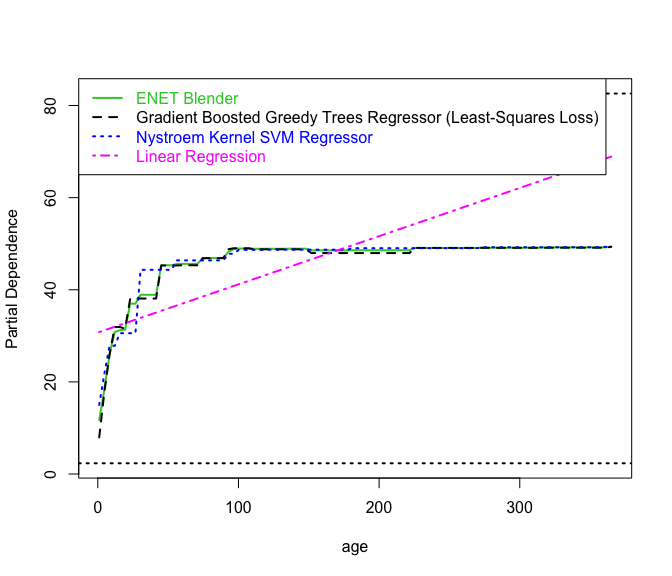Figure 3: Overlaid age partial dependence plots for four models.

Figures 3 through 6 present plots of these partial dependence functions for the variables age, cement, water, and blastFurnaceSlag, for each of the four models considered here, overlaid to facilitate comparison. Horizontal dotted black lines are drawn at the minimum and maximum values of compressive strength to provide a useful frame of reference for the variation of the functions shown in these plots. The magenta dash-dot line in Figure 3 represents the partial dependence plot for the linear regression model, and it clearly exhibits the linear behavior described in Section 3. The other three curves in Figure 3 show the partial dependence plots for the Nystroem Kernel SVM Regressor (blue dotted line), the Gradient Boosted Greedy Trees Regressor (black dashed line), and the ENET Blender (solid green line). All three of these nonlinear models exhibit hard saturation behavior, which seems intuitively reasonable - i.e., that concrete “sets” in a finite time, after which it exhibits negligible changes in physical properties. The ability of these machine learning models to capture this saturation behavior partially explains their superior prediction performance.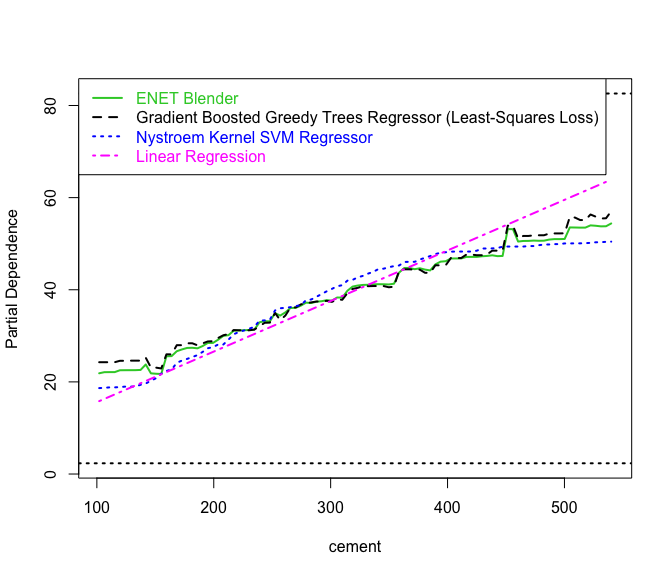Figure 4: Overlaid cement partial dependence plots for four models.

Figure 4 shows the cement partial dependence, in the same format as Figure 3. As with the age variable, the partial dependence plots for the three better models (Nystroem SVM, Gradient Boosted Greedy Trees Regressor, and ENET Blender) have similar shapes, exhibiting a much weaker nonlinearity than the age plots, but showing some evidence of saturation at both low and high cement concentrations. Also, note the substantially weaker dependence seen for these three models relative to the linear regression models.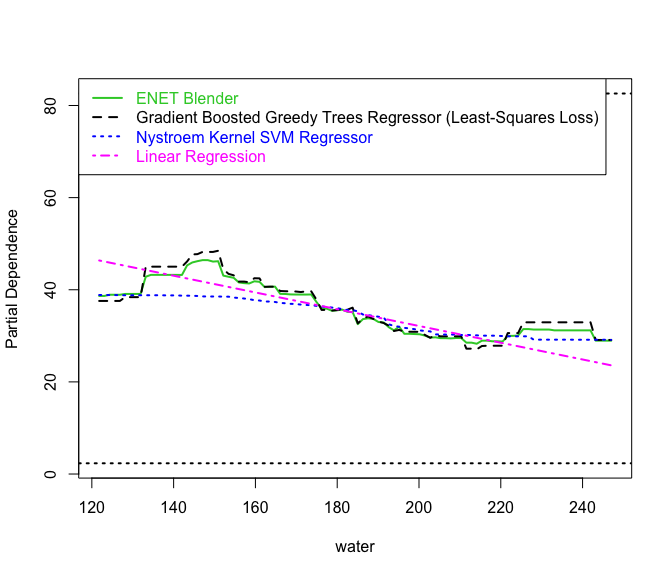Figure 5: Overlaid water partial dependence plots for four models.

Figure 5 shows the partial dependence plots for water, in the same format as Figure 3. Here, the ENET Blender and Gradient Boosted Greedy Trees Regressor exhibit nonmonotonic dependencies that are very roughly sinusoidal. The negative slope seen for the Linear Regression model is qualitatively consistent with the mid-range behavior of the ENET Blender plot, but with a substantially smaller negative slope. The plot for the Gradient Boosted Greedy Trees Regressor is extremely similar to that for the ENET blender, while the Nystroem SVM shows a very different dependence. In particular, this model shows essentially no dependence on water over the range from 120 to 180, where the ENET and GBGT models show their strongest variation.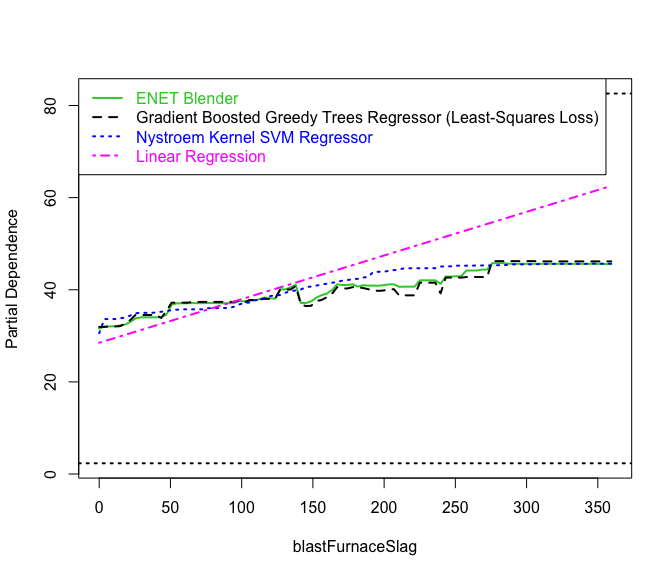Figure 6: Overlaid blastFurnaceSlag partial dependence plots for four models.

Finally, Figure 6 shows the partial dependence plots for blastFurnaceSlag, again in the same format as Figure 3. Here, all four of these plots suggest an approximately linear dependence of compressive strength on blastFurnaceSlag, all with positive slopes. The primary differences are in the magnitudes of these slopes: the linear model show much larger slopes than the other three models, all of which exhibit very similar behavior.

The results presented in Figures 3 through 6 suggest several conclusions. First, the superior predictive capability of the ENET Blender and Gradient Boosted Greedy Trees Regressor probably arises from their flexibility in capturing the monotonic saturation nonlinearity of the age dependence and the non-monotonic water dependence. Indeed, these two models are the only ones examined here that show this relatively complex water dependence. In contrast, the simple linear model can only crudely approximate the nonlinear age and water dependencies.

## 5. Summary

This note has described the partial dependence plots proposed by Friedman (2001) to characterize the dependence of model predictions on individual covariates. As discussed in Section 3, these plots reduce to straight lines for linear regression model, where the slopes correspond to the model parameters; thus, for arbitrary predictive models, these plots may be viewed as graphical extensions of the coefficients in these linear models. The other three models characterized in Figure 3 exhibit “hard saturation,” showing no influence of age beyond approximately 100 days. Finally, the most surprising feature seen in these partial dependence results is the non-monotonic water dependence shown in Figure 5 for the ENET and GBGT models: this behavior is not seen for the Nystroem Kernel SVM model, which performs clearly worse than these other models.